# Online Class: ABCs of Algebra\$60.00
no certificate
- OR -
\$85.00
with CEU Certificate*
Start Right Now!
Taking multiple courses? Save with our platinum program.

• 12
Lessons
• 24
Exams &
Assignments
• 22
Hours
average time
• 2.2
CEUs
• 1,844
Students
have taken this course## Course Description

Find out how algebra is simply a natural extension of the math you learned in elementary school. Basic Algebra is designed for all students who need a basic understanding of algebraic operations including simplifying, factoring, solving equations and inequalities, working with fractions or exponents, graphing, and word problems.

In Basic Algebra we will walk step-by-step through a wide variety of explained problems. Learn to perform the steps to solve equations and graph solutions. Understand FOIL (when it isn't wrapping your baked potato). Discover methods for factoring trinomials quickly and easily. Understand slopes and line equations so that you can identify a slope from two points or draw a line with a slope and a point. See how word problems help you translate your math skills to your real world. Explore additional resources for more explanations and practice problems with immediate feedback. Allow the course to lead you from topic to topic, building on what you already know.
• Completely Online
• Self-Paced
• 6 Months to Complete
• Start Anytime
• PC & Mac Compatible
• Android & iOS Friendly
• Accredited CEUs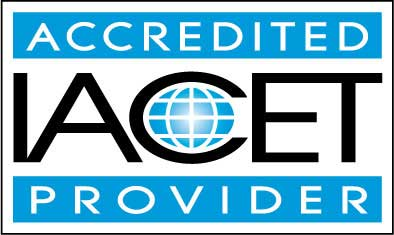## Course Lessons

Average Lesson Rating: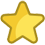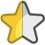#### Lesson 1 - Terminology: Words and Symbols

This lesson will cover these topics:
Numbers; Numerals, Numbers, and Digits – What's the Difference?; Equivalent, Greater Than, and Less Than (mathematical sentences: equality or inequality?); Variables and Constants; Axioms and Proper
7 Total Points
•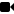Lesson 1 Video A
•Lesson 1 Video B : How to Take an Exam
•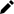Review 5 Practice Worksheets
•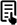Review Article: Basics of Algebra
•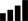Take Survey: Reasons for Taking this Course
•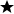Complete: Introduction Assignment
•Complete Exam: Lesson 1 Terminology: Words and Symbols

#### Lesson 2 - Properties and Principles of Equations

This lesson will cover these topics:
Special Addition and Subtraction Properties of Equations; Multiplication and Division Properties of Equations; Using the Properties to Simplify Terms in Equations, including Variables; Transformation Princip
16 Total Points
•Lesson 2 Video
•Review 5 Practice Worksheets
•Review 2 Articles: Properties of Arithmetic; Closure Property
•Complete: Lesson 2 Exercises
•Complete Exam: Lesson 2 - Properties and Principles of Equations

#### Lesson 3 - Directed Numbers in Equations

This lesson will cover these topics:
Understanding Directed (Positive and Negative) Numbers; Adding and Subtracting with Directed Numbers; Additive Inverse; Multiplying and Dividing with Directed Numbers; Multiplicative Inverse of a Number; How
14 Total Points
•Lesson 3 Video
•Review 5 Practice Worksheets
•Complete: Lesson 3 Exercises
•Complete Exam: Lesson 3 - Directed Numbers in Equations

#### Lesson 4 - Solving Problems using Equations and Inequalities

This lesson will cover these topics: Parentheses, Brackets, and Order of Operations; Simplifying an Algebraic Expression; The Steps to Solving a Problem; Problem Solving Examples; Practice Problems. 16 Total Points
•Lesson 4 Video
•Review 5 Practice Worksheets
•Review Article: Practice using FOIL
•Complete: Lesson 4 Exercises
•Complete Exam: Lesson 4 - Solving Problems using Equations and Inequalities

#### Lesson 5 - Exponents and Roots

This lesson will cover these topics:
Bases, Factors, and Exponents; Expressing Exponents in Words; Negative Exponents and Negative Bases; Adding and Subtracting Numbers with Exponents; Multiplying and Dividing Numbers with Exponents.
29 Total Points
•Lesson 5 Video
•Review 5 Practice Worksheets
•Complete Assignment: Additional Resources for Radicals and Exponents
•Complete: Lesson 5 Exercises
•Complete Exam: Lesson 5 - Exponents and Roots

#### Lesson 6 - Polynomials

Lesson topics will include:
What is a Polynomial?; Adding and Subtracting Polynomials; Multiplying and Dividing Polynomials; The Product of Powers and the Power of a Product; Uses of Polynomials – Area and Volume Problems.
20 Total Points
•Lesson 6 Video
•Review 5 Practice Worksheets
•Review 2 Articles: Polynomial division; Practice adding and subtracting polynomials
•Complete: Lesson 6 Exercises
•Complete Exam: Lesson 6 - Polynomials

#### Lesson 7 - Fractions

This lesson will cover these topics: What is a Fraction?; Proper Fractions, Improper Fractions, and Mixed Numbers; Equivalent Fractions and Lowest Terms; Greatest Common Factor and Lowest Common Denominator; Adding and Subtracting Fractions; Multiply 17 Total Points
•Lesson 7 Video
•Review 5 Practice Worksheets
•Complete: Lesson 7 Exercises
•Complete Exam: Lesson 7 - Fractions

#### Lesson 8 - Factoring to Solve Problems

This lesson will cover these topics: Common Factors; Using the Distributive Property in Graphing; Factoring the Difference of Two Squares; Squaring a Binomial 5 Total Points
•Lesson 8 Video
•Review 5 Practice Worksheets
•Complete: Lesson 8 Exam - Factoring

#### Lesson 9 - Factoring to Solve Problems (Part 2)

This lesson will cover these topics:
Combining Types of Factoring; Uses of Factoring - Examples.
15 Total Points
•Lesson 9 Video
•Review Practice Worksheet: optional-Exercise1forLesson9.pdf
•Review Article: Solve by Factoring
•Complete: Lesson 9 Exercises
•Complete Exam: Lesson 9 - Factoring to Solve Problems

#### Lesson 10 - Linear Equations and Inequalities

This lesson will cover these topics:
What Do Linear Equations Look Like?; The x and y Intercepts
15 Total Points
•Lesson 10 Video
•Review 5 Practice Worksheets
•Review 3 Articles: More help on slopes; Practice finding the slope of a line; Practice of x and y intercepts
•Complete: Lesson 10 Exercises
•Complete Exam: Lesson 10 - Linear Equations and Inequalities

#### Lesson 11 - Solving Systems of Equations

This lesson will cover these topics:
Two Variables and Two Sentences; Solving by Substitution; Solving with Addition or Subtraction to Eliminate a Variable; Solving by Graphing; Systems of Three or More Sentences.
16 Total Points
•Lesson 11 Video
•Review 5 Practice Worksheets
•Complete: Lesson 11 Exercises
•Complete Exam: Lesson 11 - Solving Systems of Equations

#### Lesson 12 - More Quadratic Equation Solution Methods

This lesson will cover these topics:
The Property of Square Roots of Equal Numbers; Completing the Trinomial Square; The Quadratic Formula; Quadratic Inequalities
227 Total Points
•Lesson 12 Video
•Review 5 Practice Worksheets
•Review 2 Articles: Practice Solving Motion Problems; Practice Solving Quadratic factoring
•Review Video: Algebra Review
•Take Poll: Final Course Poll - Your Opinion
•Take Survey: Program Evaluation Follow-up Survey (End of Course)
•Complete Exam: Lesson 12 - More Quadratic Equation Solution Methods
•Complete: The Final Exam
397
Total Course Points

## Learning Outcomes

By successfully completing this course, students will be able to:
• Identify properties and principles of equations.
• Define algebra terminology.
• Understand directed numbers in equations.
• Solve problems using equations and inequalities.
• Solve problems using exponents and roots.
• Solve polynomials.
• Solve problems involving fractions.
• Solve problems by using factoring.
• Solve linear equations and inequalities.
• Solve systems of equations.
• Demonstrate mastery of lesson content at levels of 70% or higher.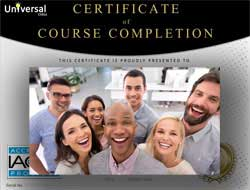• Document Your Lifelong Learning Achievements
• Earn an Official Certificate Documenting Course Hours and CEUs
• Verify Your Certificate with a Unique Serial Number OnlineCourse Title: ABCs of Algebra
Course Number: 9770476
Languages: English - United States, Canada and other English speaking countries
Category:
Course Type: General Education (Self-Paced, Online Class)
CEU Value: 2.2 IACET CEUs (Continuing Education Units)
CE Accreditation: Universal Class, Inc. has been accredited as an Authorized Provider by the International Association for Continuing Education and Training (IACET).
Assessment Method: Lesson assignments and review exams
Instructor: April Cordry-Moore
Syllabus: View Syllabus
Duration: Continuous: Enroll anytime!
Course Fee: \$60.00 (no CEU Certification) || with Online CEU Certification: \$85.00

Course Only
One Course
No Certificate / No CEUs
\$60
for 6 months

Billed once
This course only
 Includes certificate X Includes CEUs X Self-paced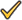Instructor supportTime to complete 6 months No. of courses 1 course
Certificate Course
One Course
Certificate & CEUs
\$85
for 6 months

Billed once
This course only
 Includes certificateIncludes CEUsSelf-pacedInstructor supportTime to complete 6 months No. of courses 1 course
Platinum YearlyALL COURSES
Certificates & CEUs
\$189
per year
You save 50%!
Billed once
Includes all 500+ courses
 Includes certificateIncludes CEUsSelf-pacedInstructor supportTime to complete 12 Months No. of courses 500+
Platinum Monthly
ALL COURSES
Certificates & CEUs
\$69 first month
\$29.00 / each consecutive month thereafter

Billed monthly
Includes all 500+ courses
 Includes certificateIncludes CEUsSelf-pacedInstructor supportTime to complete Monthly No. of courses 500+

## Student Testimonials

• "The most helpful part of the class was the exercises that were available at the end of each chapter....The instructor was great." -- Sandy E.
• "The instructor seemed knowledgeable, warm, and human.The exercises were most helpful. There is no way to learn this stuff without practice. The exercises enable true learning." -- BARBARA P.
• "Lots of practice samples available....Very helpful." -- Susan W.
• "The instructor was unbelievably available and interested in my questions and comments. Very professional and very knowledgable." -- Kate S.
• "The instructor was very helpful." -- Karsten A.
• "I couldn't have gotten a better instructor for this class. If I grade to grade her I wuld give her an A." -- LATUNIA P.